# Astronomical Distances – Parallax and the Cosmological Bean StalkAstrophysicists can identify the most distant quasars yet discovered by science.  But how can you determine astronomical distances?  And how do cosmologists actually know how far away astronomical objects are?

World views have been changing ever since us, human beings, became self-aware and started looking up at the sky…

Centuries ago, natural philosophers still thought that our Galaxy, the Milky Way, was the entire extent of our Universe.

But…  Just HOW do astronomers measure the distance to stars and galaxies exactly?

## Using Direct Distance Measurement

Astronomical distances to celestial bodies are not always easy to determine.  Even with precision space-based telescopes, direct distance measurements are only possible for stars within a distance of little more than 1,000 light years away from Earth.

Astronomers use parallax – a phenomenon that is familiar to almost everyone in everyday life.

# Parallax: A Shift in Perspective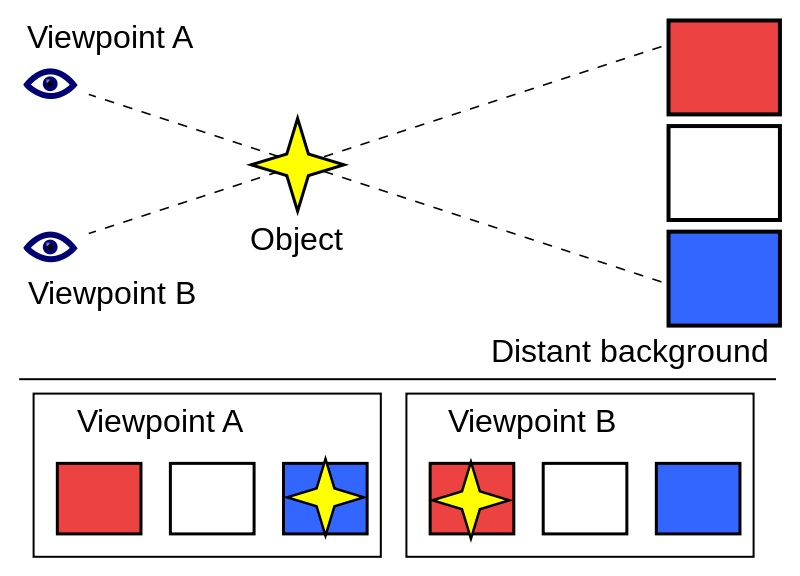When viewed from “Viewpoint A” (Left Eye), the object appears to be in front of the blue square. When the viewpoint is changed to “Viewpoint B” (Right Eye), the object appears to have moved in front of the red square. Source: Wikipedia

Parallax is a shift in perspective – the difference in apparent position of an object viewed along two different lines of sight.

Objects appear to be in slightly different positions against their background when viewed solely through the left eye compared to when they are viewed solely through the right eye.

As human eyes, and those of other animals, are usually located in different positions on the head, they present different views simultaneously.

As Nature goes, the human brain uses this information to achieve a mighty clever trick

### Depth Perception

The brain uses parallax to estimate the distance of an object.

The parallax is measured by the angle or semi-angle of inclination between those two lines of sight.

##### Parallax is used to determine distances.

Due to perspective, nearby objects show a larger parallax than farther objects when observed from different positions.

This is the basis of stereopsis – the process by which the brain exploits the phenomenon of parallax.

Cleverly, the human brain uses the separation between the two eyes to generate a perception of depth, and estimate the distance to an object automatically.

If your vision happens to be monocular only, then it is most difficult to determine whether an object is either small or far away.

However, in the majority of cases, the closer the object, the larger the parallax.

The main point is…

# Using Stellar Parallax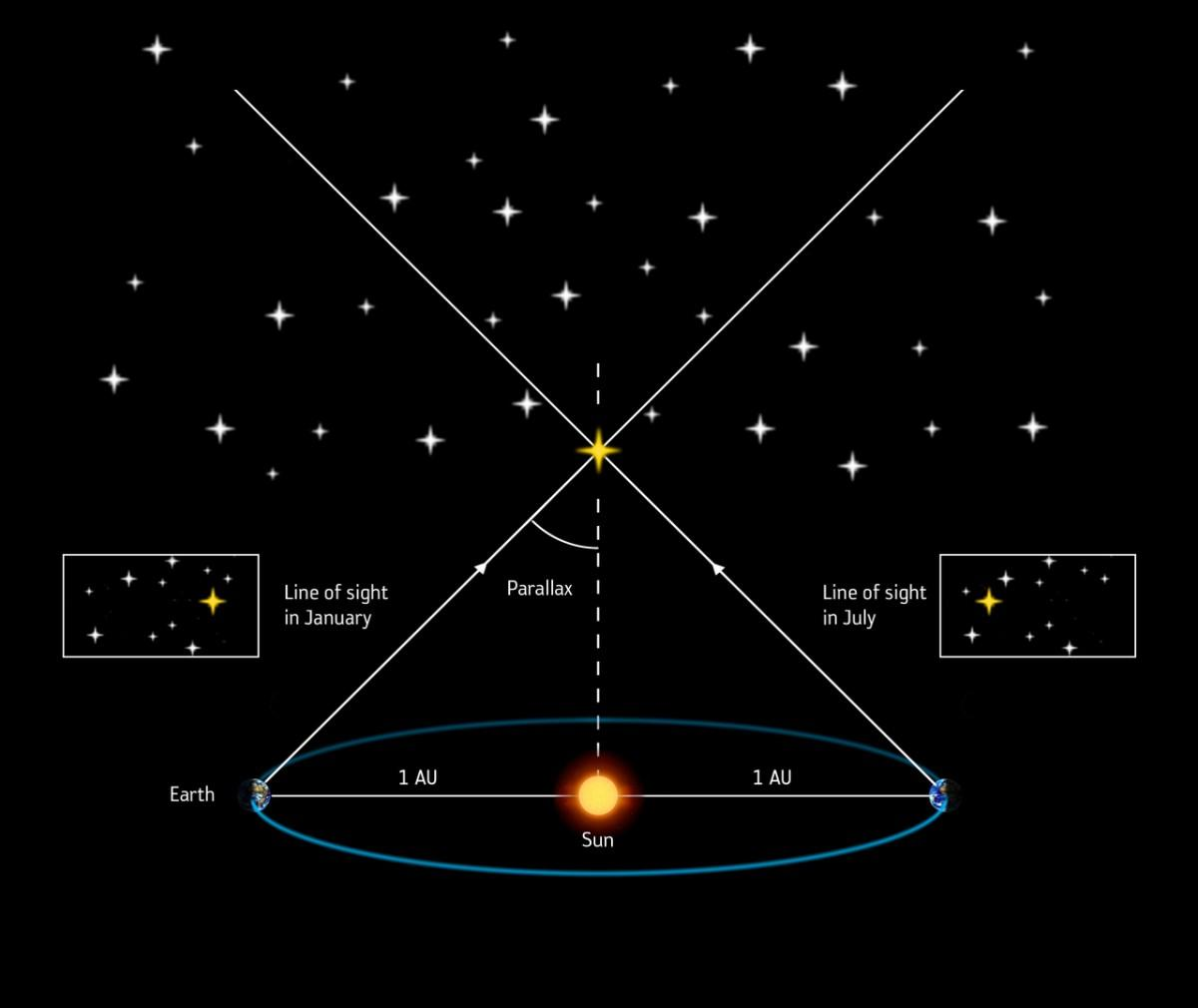The same principle can be used to work out the distance to stars, accurately and mathematically, rather than automatically.

Over the course of a year, the Earth orbits the Sun.

The apparent position of comparatively nearby stars changes relative to much more distant ones, which are so far away that they appear never to move.

## Parallax Angle

The parallax angle by which the apparent position changes is inversely proportional to the star’s distance.$distance (parsecs) = \frac{1}{parallax(arcseconds)}$

### Distance = Reciprocal of Angle

Assuming the angle is small, the distance d to the object (in parsecs) is the reciprocal of the parallax (in arcseconds):$\frac{1}{p(arcsec)} = d (pc)$

For example, the distance to Proxima Centauri is$\frac{1}{0.7687} = 1.3009 parsecs (4.243 ly)$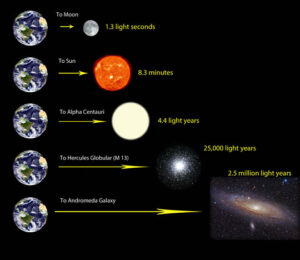From the Moon in our close neighbourhood, all the way out to the Andromeda Galaxy, the most distant celestial object visible to the naked eye from Earth, this scale gives an appreciation of astronomical distances. Source: Sky and Telescope.

This method works well to calculate the distances of close celestial objects like the Moon and the Sun, up to objects approximately 1,000 light years away.

The stellar parallax method also defines the unit of distance, i.e. the parsec.

### The Parsec Distance Unit

The nearest star to the Sun is Proxima Centauri around 1.3 parsecs (or 4.2 light-years) away.

 1 parsec (pc) ≈ 206264.806247096 astronomical units (AU) ≈ 3.085677581×1016 metres (m) ≈ 30.856775815 trillion kilometres (km) ≈ 19.173511577 trillion miles ≈ 3.261563777 light-years (ly)

#### First Parallax MeasurementThe first successful measurement of the distance to a star outside our solar system using this stellar parallax method was carried out by the German astronomer Friedrich Bassel in 1838, when he determined that 61 Cygni is 10.4 ly away.  More accurate recent measurements give a distance of 11.4 ly.  About 3.48 pc.

## Using Standard Candles and Magnitudes

While parallax is used to calibrate the astronomical distance scale by allowing us to work out the distances to nearby stars, other methods must be used for much more cosmologically distant bodies, since their parallax angle is too small to measure accurately.

One comes from using the inherent brightness of an object.

### Apparent magnitude vs Absolute Magnitude

The luminosity of some astronomical bodies is sufficiently well-known well enough to be able to calculate their distance.

Since how bright a star appears in the sky, its apparent magnitude m is a function of both its actual light output (or absolute magnitude M) and the astronomical distance from the observer to the star.$m = M + 5log (d) - 5$

Knowing the former two values allows calculation of the latter.$distance = 10^{\frac{(m-M+5)}{5}}$

The bodies of which the intrinsic luminosity is well known are referred to as “standard candles”.

## Cepheid Variable Stars

One commonly used type of standard candle is the Cepheid variable star – named after Delta Cephei in the constellation Cepheus.

Cepheid variables are pulsating stars that brighten and fade over time in a predictable pattern.

Because there is a direct relationship between its luminosity and the period over which it fluctuates, the absolute magnitude of any Cepheid variable, close enough to see, can be worked out.

#### M31-V1 – The Star that Changed the UniverseNASA’s Hubble Space Telescope has been trained on a single variable star that in 1923 altered the course of modern astronomy. V1 is a special class of pulsating star called a Cepheid variable that can be used to make reliable measurements of large cosmic distances. Credit: NASA, ESA, and the Hubble Heritage Team (STScI/AURA)

The identification of this type of star in the Andromeda galaxy, and observations by Edwin Hubble in 1922–1923 – a century ago – led astronomers to realise for the first time that Andromeda was much further away outside our own  galaxy than had been thought!The illustrated graph shows that V1 completes a pulsation cycle every 31.4 days. The red dots on the graph represent observations by the American Association of Variable Star Observers (AAVSO), who partnered with the Space Telescope Science Institute’s Hubble Heritage Project to study the star. The four stars on the graph denote observations made by the Hubble Space Telescope’s Wide Field Camera 3. Ten amateur astronomers from around the world made 214 observations of V1 between July 2010 and December 2010, obtaining four pulsation cycles. The AAVSO study allowed the Hubble Heritage team to target Hubble observations that would capture the star at its brightest and dimmest phases.

Astronomical distances calculated using variable stars as standard candles were recalculated after Walter Baade’s observations in the 1950s revealed that the more distant Cepheids (Type II) were older and had a lower non-hydrogen content than ones nearby.

Their absolute magnitudes had been underestimated, and the Cepheids were much further away than had been believed when they were first identified as extragalactic.

## RR Lyrae Variables and Type 1a Supernovae

At greater distances yet, or for areas in which there is no star formation and hence few Cepheids, such as in elliptical galaxies, other astronomical bodies are used to calculate distances in a similar way.

These might be RR Lyrae variables (a type of red giant star) or Type 1a supernovae, for example.

### RR Lyrae

RR Lyrae variables serve as important standard candles that are used to measure astronomical distances.

The period of pulsation of an RR Lyra variable star depends on its mass, luminosity and temperature, while the difference between the measured luminosity and the actual luminosity allows its distance to be determined via the inverse-square law.

### The Inverse Square LawThe Inverse Square Law, where S represents a light source, while r represents the measured points. The lines represent the flux emanating from the sources and fluxes. Source: Wikipedia

For binary systems, the orbital characteristics can be used to calculate mass, and, since there is a direct relationship between mass and luminosity – the absolute magnitude of a star.$intensity \propto \frac{1}{distance^2}$

Hence the distance to the object can be inferred.

### Type 1-A Supernovae

Meanwhile, a long time ago in a galaxy far, far away…

Type 1a category supernovae most often occur in binary systems.

These supernovae produce fairly consistent peak luminosities because of the fixed critical mass at which a white dwarf will explode.

Their consistent peak luminosity allows these stellar explosions to be used as standard candles to measure the distance to their host galaxies.

# Finding the Distances to Galaxies

Other astrophysical formulae can also be used to determine absolute magnitude, and therefore distance:

• the Tully-Fisher relation is aThe Tully–Fisher relation for spiral and lenticular galaxies.  Source: Wikipedia

correlation between a spiral or lenticular galaxy’s rotational velocity$v_{rot}$ and its luminosity that, along with the galaxy’s apparent magnitude, can used to establish the distance to it.$L \propto v_{rot}$

the Faber-Jackson relation provided the first empirical power-law relation between the luminosity and the central stellar velocity dispersion σ of an elliptical galaxy.$L \propto \sigma^4$

# A Redshift in Trigonometric ParallaxAs well as realising that the Andromeda Galaxy is separate from our own, Edwin Hubble discovered that the redshift of light from other galaxies is proportional to how far away they are from us.

This is now known as Hubble’s law:$v = H_0 d$

where$v = recessional \: velocity \: of \: galaxy$$H_0=Hubble \: constant$$d = distance \: to \: galaxy$

### Galaxy Recession Velocity

The large redshifts of the light from what we now know to be distant galaxies, were first noted by the American astronomer Vesto Slipher in 1912, and are a result of the Doppler Effect:$v_r = c \times \frac {\delta \lambda}{\lambda_0}$

where$c = speed \: of \: light$$\delta \lambda = change \: in \: wavelength$$\lambda_0 = rest \: wavelength$

The magnitude of the shift depends on the relative speed of motion between the source and the observer.

##### The higher the speed, the larger the shift.

Galaxies further from the Earth are moving away from it faster than ones close by.

Of course, Hubble had massively overestimated the rate at which galaxies’ recession velocities increase with distance.

This happened because of the error in calibrating those distances that came from confusing the two types of Cepheid variables, as mentioned above.

Increasingly precise measurements of this particular number – the Hubble’s constant – have been made from Allan Sandage’s 1958 first reasonable estimate onwards.

In 2010, data from the WMAP satellite gave us a value of$H_0 \approx 71.0 \pm 2.5 (\frac{km}{s})Mpc^{-1}$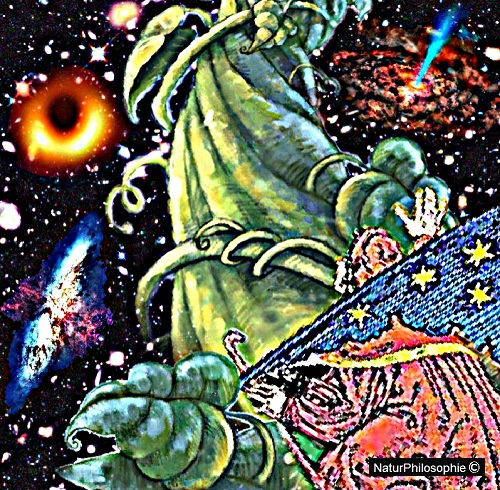For the most distant bodies in the universe, corrections have to be made to take general relativity into account.

A discovered quasar with a redshift of 7.085, dating it to just 0.77 billion years after the Big Bang, places it about 13.35 billion light years away from Earth.

## How fast is the Universe expanding?

The Hubble constant is one of the most important numbers in cosmology because it tells us how fast the universe is expanding, and it can be used to determine the age of the universe and its history.

The Hubble constant H0 is most frequently quoted in km s-1 Mpc-1, thus giving the speed in km/s of a galaxy located 1 Megaparsec (or 3.09×1019 km) away.

But the Hubble constant can also be interpreted as the relative rate of expansion of the Universe.

In this form, the constant is$H_0 \approx 7 \% Gyr^{-1}$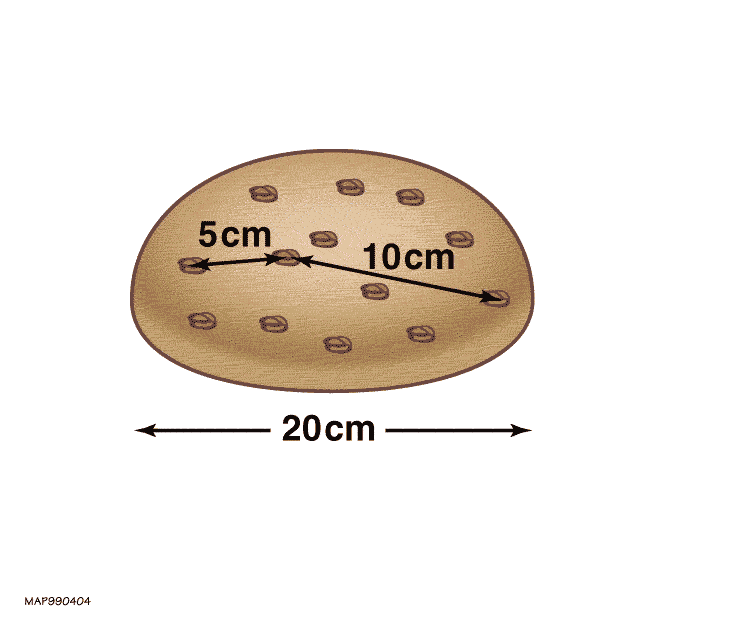H0 = 7%/Gyr, meaning that at the current rate of expansion it takes a billion years for an unbound structure to grow by 7%.

Before I leave you on that thought, here is just one other thing to contemplate…